Courses

# Test: Long And Short - 1

## 10 Questions MCQ Test Mathematics (Maths) for Class 4 | Test: Long And Short - 1

Description
This mock test of Test: Long And Short - 1 for Class 4 helps you for every Class 4 entrance exam. This contains 10 Multiple Choice Questions for Class 4 Test: Long And Short - 1 (mcq) to study with solutions a complete question bank. The solved questions answers in this Test: Long And Short - 1 quiz give you a good mix of easy questions and tough questions. Class 4 students definitely take this Test: Long And Short - 1 exercise for a better result in the exam. You can find other Test: Long And Short - 1 extra questions, long questions & short questions for Class 4 on EduRev as well by searching above.
QUESTION: 1

### How long is the pencil according to the ruler?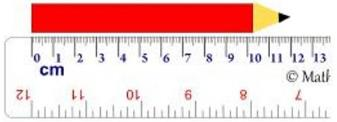Solution:

cm means centimeters
The pencil starts at 0, and ends at the 12 cm mark.
So it is 12 cm long.

QUESTION: 2

### How long is the knife according to the ruler?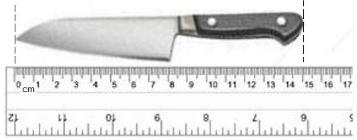Solution:

cm means centimeters
The knife starts at 0, and ends at the 15 cm mark.
So it is 15 cm long.

QUESTION: 3

### What is the width of the rectangle according to the rulers?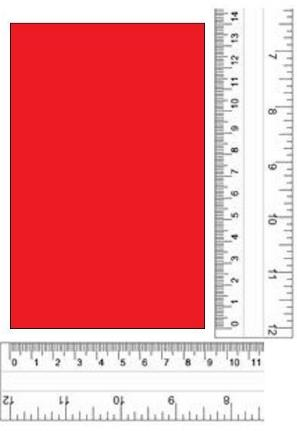Solution:

"cm" means centimeters
Use the horizontal ruler.
The width of the rectangle starts at 0 cm and ends at the 9 cm mark.
So its width is 9 cm.

QUESTION: 4

How long is the fence according to the measuring tape?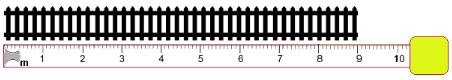Solution:

m means meters
The fence starts at 0, and ends at the 9 m mark.
So it is 9 m long.

QUESTION: 5

How long is the bus according to the measuring tapes?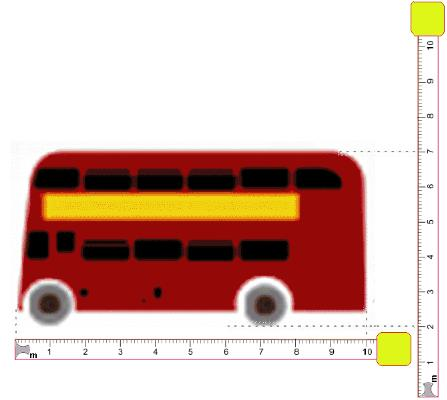Solution:

m means meters
The front of the bus starts at 0, and the back of the bus is at the 10 m mark.
So it is 10 m long.

QUESTION: 6

What is the height of the rectangle according to the rulers?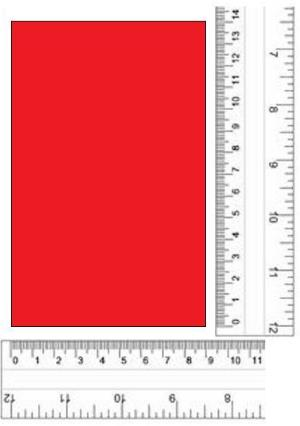Solution:

"cm" means centimeters.
Use the vertical ruler.
The height of the rectangle starts at 0 cm and ends at the 14 cm mark.
So its height is 14 cm.

QUESTION: 7

How long is the fence according to the measuring tape?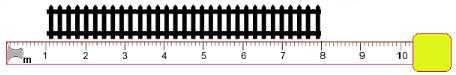Solution:

m means meters
The fence starts at 1 m and ends at the 8 m mark.
8 − 1 = 7
So it is 7 m long.

QUESTION: 8

How long is the spoon according to the ruler?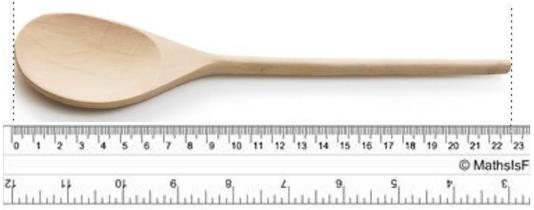Solution:

"cm" means centimeters.
The spoon starts at 0 cm, and ends at the 23 cm mark.
So it is 23 cm long.

QUESTION: 9

How long is the spoon according to the ruler?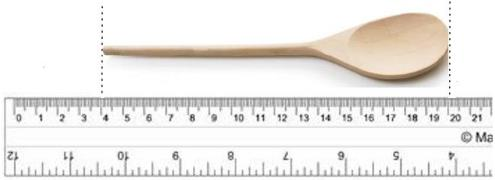Solution:

"cm" means centimeters.
The spoon starts at 4 cm, and ends at the 20 cm mark.
20 - 4 = 16
So it is 16 cm long.

QUESTION: 10

How high is the balloon and basket according to the measuring tapes?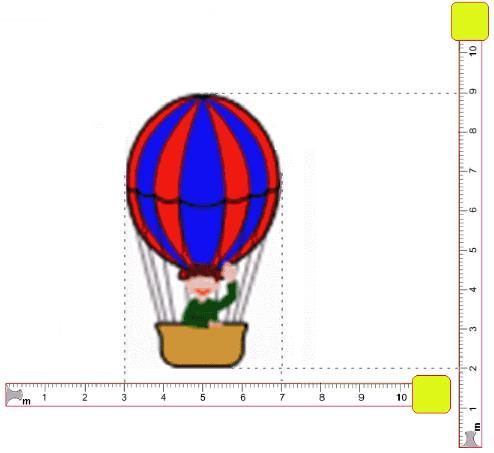Solution:

m means meters
The balloon and basket height starts at 2 m, and ends at the 9 m mark.
9 − 2 = 7
So it is 7 m high.